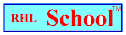Name_______________________________________________Date_________________________
Mathematics Problem Solving
Volume 4, Number 24, March 15, 1999
www.rhlschool.com

What Would You Do?

What would, or more precisely, what should you do to solve the following problems?

1. Pablo ran in an eleven mile race. A mile equals 5,280 feet. How many feet did Pablo run in his race?

a. Divide 5,280 by 11.
b. Multiply 11 times 5,280.
d. Subtract 11 from 5,280.

2. Kristy has a jar that contains 43,065 gumballs. She wants to put all the gumballs into 87 small jars. She wants to put exactly the same number of gumballs into each jar. How many gumballs will she put into each jar?

a. Subtract 87 from 43,065.
b. Divide 43,065 by 87.
c. Chew all the gumballs.
d. Multiply 87 times 43,065.

3. Andrea weighs 145 pounds. She is pleased because just a month ago she weighed 153 pounds. How much weight ( in pounds) did she lose since last month?

a. Subtract 145 from 153.
b. Subtract the amount she lost from 153.
d. Divide 153 by 145.

4. These are Brian’s pets: 16 cats, 24 dogs, and 2 parrots. How many pets does Brian have?

a. Add 16 and 24; then divide that sum by 2.
b. Add 16 plus 24 plus 2.
c. Multiply 16 times the sum of 24 and 2.
d. Subtract 16 from 24 and then subtract 2 more.

5. Johnny is very proud of his new Website. It had 862 visitors in the first week it was online. It had 1,991 visitors in the second week, and 2,406 visitors in the third week. In the first three weeks, Johnny’s site had an average of how many visitors per day?

a. Add 862 plus 1, 991 plus 2,406 and divide it by 3.
b. Add 862 plus 1, 991 plus 2,406 and subtract 3.
c. Add 862 plus 1, 991 plus 2,406 plus 3.
d. Add 862 plus 1, 991 plus 2,406 and divide it by the product of 3 and 7.

1________2________3________4________5________